You are not logged in.Heatmap erstellen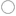Feanor

Master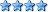•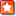Posts: 2,744

Location: Muenchen

Occupation: GER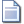Thursday, February 17th 2011, 3:36pm

Heatmap erstellen

Ich möchte eine Heatmap erstellen, pber die Verteilug meiner Zellen auf einer 500 x 500 um großen Fläche.
Ich habe die XY koordinaten meiner Zellen. Ich habe leider kA welche Software dazu am besten ist.sylencePosts: 1,855

Location: Dresden

Occupation: GERThursday, February 17th 2011, 3:56pm

z.B. gnuplot (Beispiel)El_Marinero

MasterPosts: 2,606

Occupation: GERThursday, February 17th 2011, 3:56pm

Entweder Matlab/Octave, was gnuplot ruft oder aber R.GWC_Vegeta

MasterPosts: 2,153

Location: Freiberg

Occupation: GERThursday, February 17th 2011, 5:42pm

MinecraftFeanor

Master•Posts: 2,744

Location: Muenchen

Occupation: GERSaturday, February 19th 2011, 12:03am

Okay vielen Dank,

ich habe mich für Matlab entschieden, da es bereits auf den PCs bei uns installiert ist.
Ich muss ehrlich sagne, dass programm überfordert meine Fähigkeiten auf diesem Gebiet komplett.
Ich habe es jetzt geschafft Testweise einen Scatter Plot mit Hilfe einer Bsp. XY koordinaten zu erstellen.

So nun habe ich ein Skript gefunden, was mir die Abweichungen der density des Plots anzeigt.
http://www.mathworks.com/matlabcentral/f…52-smoothhist2d

Nur wie ich das und meinen Plot zusammen bekomme ist mir ein komplettes Rätsel, ich habe mir den Code länger angeschaut, aber verstehe es einfach nicht mit meinen rudimentären Wissen.
Ich würde mich sehr freuen, wenn mir jmd helfen könnte.

danke

PS: Wenn es jmd schafft mir zu vermitteln wie ich dies vernüftig und attraktiv darstellen kann, würde ich eine Kiste bier springen lassen

This post has been edited 1 times, last edit by "Feanor" (Feb 19th 2011, 12:08am)CF_Ragnarok

Sage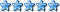Posts: 12,971

Occupation: AUTSaturday, February 19th 2011, 12:21am

hab das da als e-book pdf am pc...kanns dir bei bedarf mal wo hochladen_Icedragon_

MasterPosts: 2,868

Location: Kloster

Occupation: StatistikerSaturday, February 19th 2011, 9:16am

sry der beitrag war schwachsinn

This post has been edited 2 times, last edit by "_Icedragon_" (Feb 19th 2011, 10:45am)Feanor

Master•Posts: 2,744

Location: Muenchen

Occupation: GERSaturday, February 19th 2011, 10:04am

muss ich dann 10k werte manual eintragen ?

Ragna das wäre sehr nice

An Welcher stelle kann ich dort überhaupt die Daten einlesen lassen ?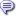Quoted

function smoothhist2D(X,lambda,nbins,outliercutoff,plottype)
% SMOOTHHIST2D Plot a smoothed histogram of bivariate data.
% SMOOTHHIST2D(X,LAMBDA,NBINS) plots a smoothed histogram of the bivariate
% data in the N-by-2 matrix X. Rows of X correspond to observations. The
% first column of X corresponds to the horizontal axis of the figure, the
% second to the vertical. LAMBDA is a positive scalar smoothing parameter;
% higher values lead to more smoothing, values close to zero lead to a plot
% that is essentially just the raw data. NBINS is a two-element vector
% that determines the number of histogram bins in the horizontal and
% vertical directions.
%
% SMOOTHHIST2D(X,LAMBDA,NBINS,CUTOFF) plots outliers in the data as points
% overlaid on the smoothed histogram. Outliers are defined as points in
% regions where the smoothed density is less than (100*CUTOFF)% of the
% maximum density.
%
% SMOOTHHIST2D(X,LAMBDA,NBINS,[],'surf') plots a smoothed histogram as a
% surface plot. SMOOTHHIST2D ignores the CUTOFF input in this case, and
% the surface plot does not include outliers.
%
% SMOOTHHIST2D(X,LAMBDA,NBINS,CUTOFF,'image') plots the histogram as an
% image plot, the default.
%
% Example:
% X = [mvnrnd([0 5], [3 0; 0 3], 2000);
% mvnrnd([0 8], [1 0; 0 5], 2000);
% mvnrnd([3 5], [5 0; 0 1], 2000)];
% smoothhist2D(X,5,[100, 100],.05);
% smoothhist2D(X,5,[100, 100],[],'surf');
%
% Reference:
% Eilers, P.H.C. and Goeman, J.J (2004) "Enhancing scaterplots with
% smoothed densities", Bioinformatics 20(5):623-628.

% Copyright 2009 The MathWorks, Inc.
% Revision: 1.0 Date: 2006/12/12
%
% Requires MATLAB&#65533; R14.

if nargin < 4 || isempty(outliercutoff), outliercutoff = .05; end
if nargin < 5, plottype = 'image'; end

minx = min(X,[],1);
maxx = max(X,[],1);
edges1 = linspace(minx(1), maxx(1), nbins(1)+1);
ctrs1 = edges1(1:end-1) + .5*diff(edges1);
edges1 = [-Inf edges1(2:end-1) Inf];
edges2 = linspace(minx(2), maxx(2), nbins(2)+1);
ctrs2 = edges2(1:end-1) + .5*diff(edges2);
edges2 = [-Inf edges2(2:end-1) Inf];

[n,p] = size(X);
bin = zeros(n,2);
% Reverse the columns of H to put the first column of X along the
% horizontal axis, the second along the vertical.
[dum,bin(:,2)] = histc(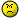:,1),edges1);
[dum,bin(:,1)] = histc(:,2),edges2);
H = accumarray(bin,1,nbins([2 1])) ./ n;

% Eiler's 1D smooth, twice
G = smooth1D(H,lambda);
F = smooth1D(G',lambda)';
% % An alternative, using filter2. However, lambda means totally different
% % things in this case: for smooth1D, it is a smoothness penalty parameter,
% % while for filter2D, it is a window halfwidth
% F = filter2D(H,lambda);

relF = F./max(F(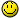);
if outliercutoff > 0
outliers = (relF(nbins(2)*(bin(:,2)-1)+bin(:,1)) < outliercutoff);
end

nc = 256;
colormap(hot(nc));
switch plottype
case 'surf'
surf(ctrs1,ctrs2,F,'edgealpha',0);
case 'image'
image(ctrs1,ctrs2,floor(nc.*relF) + 1);
hold on
% plot the outliers
if outliercutoff > 0
plot(outliers,1),outliers,2),'.','MarkerEdgeColor',[.8 .8 .8]);
end
% % plot a subsample of the data
% Xsample =randsample(n,n/10),;
% plot(Xsample(:,1),Xsample(:,2),'bo');
hold off
end

%-----------------------------------------------------------------------------
function Z = smooth1D(Y,lambda)
[m,n] = size(Y);
E = eye(m);
D1 = diff(E,1);
D2 = diff(D1,1);
P = lambda.^2 .* D2'*D2 + 2.*lambda .* D1'*D1;
Z = (E + P) \ Y;
% This is a better solution, but takes a bit longer for n and m large
% opts.RECT = true;
% D1 = [diff(E,1); zeros(1,n)];
% D2 = [diff(D1,1); zeros(1,n)];
% Z = linsolve([E; 2.*sqrt(lambda).*D1; lambda.*D2],[Y; zeros(2*m,n)],opts);

%-----------------------------------------------------------------------------
function Z = filter2D(Y,bw)
z = -1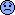1/bw):1;
k = .75 * (1 - z.^2); % epanechnikov-like weights
k = k ./ sum(k);
Z = filter2(k'*k,Y);

This post has been edited 2 times, last edit by "Feanor" (Feb 19th 2011, 10:42am)CF_Ragnarok

SagePosts: 12,971

Occupation: AUTSaturday, February 19th 2011, 10:42am

hm wär wohl besser per pm, aber nachdem du das deaktiviert hast...there you go

€: ich würde aus deinem code mal die ganzen smilies raustun...is ja klar, dass da nix funktioniert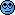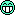This post has been edited 1 times, last edit by "CF_Ragnarok" (Feb 19th 2011, 10:43am)GWC_Vegeta

MasterPosts: 2,153

Location: Freiberg

Occupation: GERSaturday, February 19th 2011, 10:44am

also in matlab gibts da einfach den Befehl heatmap(DataMatrix,...)

[X,Y] = meshgrid(-10000:50:10000,10000:-50:-10000);
>> XY = (X.^2 + Y.^2).^0.5;
heatmap(XY);

Wobei du nur für XY deine datei einladen musst.

Kannst es aber auch noch über hot(...) machen:

image(rgb);
im = mean(rgb,3);
image(im);
colormap(hot(256))Feanor

Master•Posts: 2,744

Location: Muenchen

Occupation: GERSaturday, February 19th 2011, 10:52am

Vielen Dank Ragna

Vegeta, das sieht ja gar nicht so kompliziert aus.

Also moment sind meine Daten noch in Excel tabelle. Die kann ich doch mit MatLab einlesen lassen.

Also Mittlerweile werden die Daten die Daten eingelesen

>> [X,Y] = meshgrid(-10000:50:10000,10000:-50:-10000);
XY = (X.^2 + Y.^2).^0.5;
heatmap(data)
HeatMap object with 54 rows and 2 columns.

ich möchte aber nicht 2 columns, sondern dass die in einem X Y Koordinaten System geplotet werden, und der mir die Dichte mit HIlfe einer Heatmap anzeigt

This post has been edited 2 times, last edit by "Feanor" (Feb 19th 2011, 2:13pm)